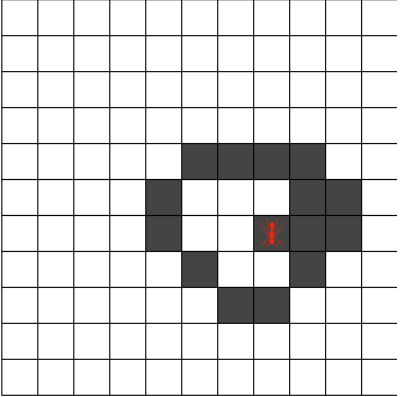# 蓝桥历届真题：兰顿蚂蚁兰顿蚂蚁，是于1986年，由克里斯·兰顿提出来的，属于细胞自动机的一种。

蚂蚁的头部朝向为：上下左右其中一方。

若蚂蚁在黑格，右转90度，将该格改为白格，并向前移一格；
若蚂蚁在白格，左转90度，将该格改为黑格，并向前移一格。

输入数据的第一行是 m n 两个整数（3 < m, n < 100），表示正方形格子的行数和列数。
接下来是 m 行数据。
每行数据为 n 个被空格分开的数字。0 表示白格，1 表示黑格。

输出数据为两个空格分开的整数 p q, 分别表示蚂蚁在k步后，所处格子的行号和列号。

5 6
0 0 0 0 0 0
0 0 0 0 0 0
0 0 1 0 0 0
0 0 0 0 0 0
0 0 0 0 0 0
2 3 L 5

1 3

3 3
0 0 0
1 1 1
1 1 1
1 1 U 6

0 0

import java.util.Scanner;

public class Main {
public static void main(String[] args) {
// TODO Auto-generated method stub
Scanner sc=new Scanner(System.in);
int m=sc.nextInt();
int n=sc.nextInt();
int[][] g=new int[m][n];
for (int i = 0; i <m; i++) {
for (int j = 0; j <n; j++) {
g[i][j]=sc.nextInt();
}
}
int x=sc.nextInt();
int y=sc.nextInt();
String s=sc.next();
int k=sc.nextInt();
int step=0;//计数
int d=getD(s);//初始的方向
while (true) {
//若蚂蚁在黑格，右转90度，将该格改为白格，并向前移一格；
if (g[x][y]==1) {
d=d%4+1;
g[x][y]=0;
}
//　若蚂蚁在白格，左转90度，将该格改为黑格，并向前移一格。
else {
d--;
if(d==0)d=4;
g[x][y]=1;
}
//前进一步
if(d==1)x--;
if(d==2)y++;
if(d==3)x++;
if(d==4)y--;
step++;
if (step==k) {
System.out.println(x+" "+y);
break;
}
}

}
//声明一个函数来获取方向
static int getD(String s){
if (s.equals("U")) return 1;
if (s.equals("R")) return 2;
if (s.equals("D")) return 3;
if (s.equals("L")) return 4;
return 0;
}
}©️2019 CSDN 皮肤主题: 1024 设计师: 上身试试